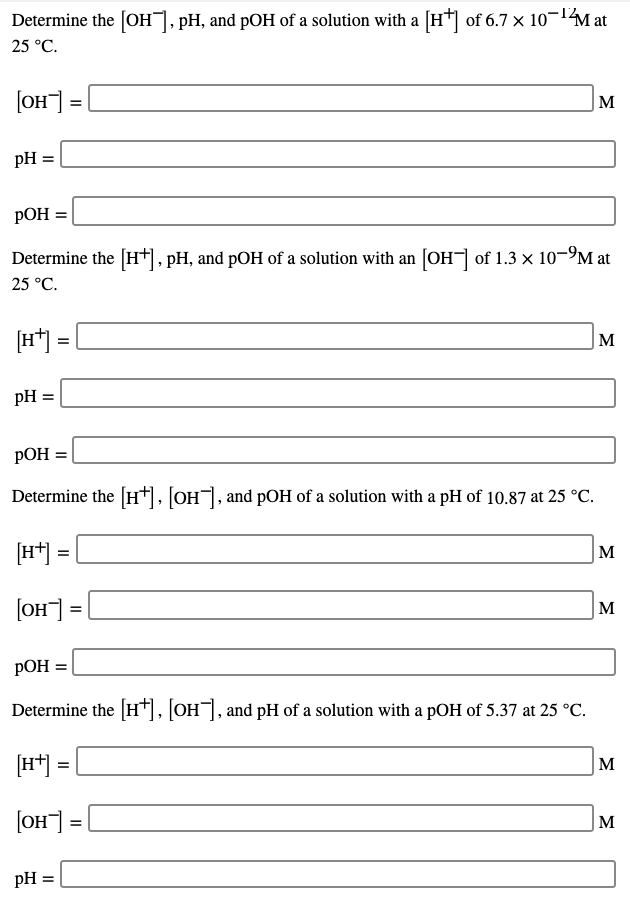# Determine the [OH], pH, and pOH of a solution with a [H of 6.7 x 10-1Mat 25 °C. [он] м pH : РОН %3 Determine the [H+], pH, and pOH of a solution with an [OH of 1.3 × 10-9M at 25 °C. [H*] = м pH : РОН %3 Determine the [H*], [OH], and pOH of a solution with a pH of 10.87 at 25 °C. [H*] = |м (он] м РОН %3 Determine the H*], |OH], and pH of a solution with a pOH of 5.37 at 25 °C. [H*) = |м |он] - M pH =

Questionhelp_outlineImage TranscriptioncloseDetermine the [OH], pH, and pOH of a solution with a [H of 6.7 x 10-1Mat 25 °C. [он] м pH : РОН %3 Determine the [H+], pH, and pOH of a solution with an [OH of 1.3 × 10-9M at 25 °C. [H*] = м pH : РОН %3 Determine the [H*], [OH], and pOH of a solution with a pH of 10.87 at 25 °C. [H*] = |м (он] м РОН %3 Determine the H*], |OH], and pH of a solution with a pOH of 5.37 at 25 °C. [H*) = |м |он] - M pH = fullscreen

### Want to see this answer and more?

Experts are waiting 24/7 to provide step-by-step solutions in as fast as 30 minutes!*

*Response times may vary by subject and question complexity. Median response time is 34 minutes for paid subscribers and may be longer for promotional offers.
Tagged in
Science
Chemistry

### Equilibrium Concepts